Multivariable Calculus: Mathematica Review

AScalarFunction is defined everywhere in (x,y,z)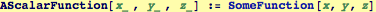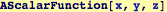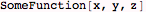The following lines print and they define expressions.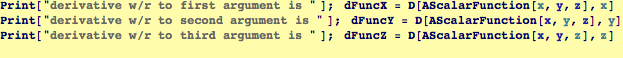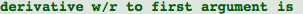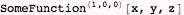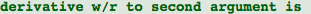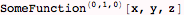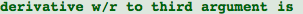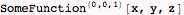In the output lines above, partial derivatives are denoted by superscripts: e.g., (1,0,0) indicates the first partial derivative with respect to the first variable, x. The second partial with respect to y and z would be denoted by a superscript (0,1,1).

x(w,v), y(w,v), z(w,v) is a restriction of all space to a surface parameterized by (w,v),
AScalarFunction is now defined on the surface as a function of (w,v)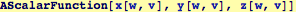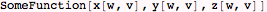Because it is now a function of w and v, the derivative with respect to x will vanish: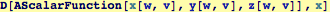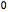Two more flavors of derivatives, these are partial derivatives evaluated on the surface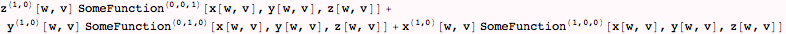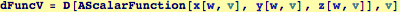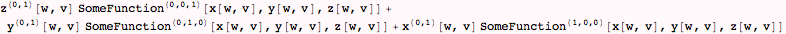On the surface x(w,v), y(w,v), z(w,v), we can prescribe a curve w(t), v(t), now we have
AScalarFunction defined on that curve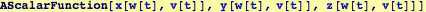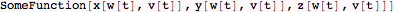The following is a derivative of the function along the curve parameterized by t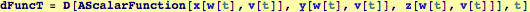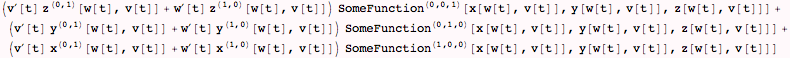Note on the step immediately above: by specifying w and v, values of x and y are specified, and additionally values of z.  The three functions x(w,v), y(w,v), and z(w,v) together describe a surface---it specifies that three points can be specified by two values (A familiar case is when w=x, and v=y, then z(x,y) is a surface that can be specified over the x-y plane. The functions w(t) and v(t) trace out a plane curve on the w-v surface and map onto a corresponding twisted curve (see Kreyszig p.429 for distinction between "plane" and "twisted" if it is not obvious).

Finally, we could skip the surface and just define a space curve x(t), y(t), z(t) and  take the derivative of AScalarFunc along  that curve: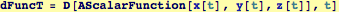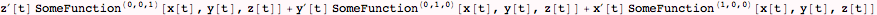Created by Wolfram Mathematica 6.0  (20 September 2007)